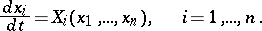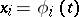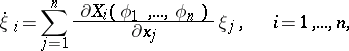# Andronov-Witt theorem

(diff) ← Older revision | Latest revision (diff) | Newer revision → (diff)

A modification of Lyapunov's theorem (on the stability of a periodic solution of a non-autonomous system of differential equations) for the autonomous system(1)

Let(2)

be a periodic solution of the system (1), and let(3)

be the corresponding system of variational equations which always has, in the case here considered, one zero characteristic exponent. The Andronov–Witt theorem is then valid: Ifcharacteristic exponents of the system (3) have negative real parts, a periodic solution (2) of the system (1) is stable according to Lyapunov (cf. Lyapunov characteristic exponent; Lyapunov stability).

The Andronov–Witt theorem was first formulated by A.A. Andronov and A.A. Witt in 1930 and was proved by them in 1933 .